In :
from __future__ import division
from numpy import *
%pylab inline
style.use('bmh')
cols = ['#348ABD', '#A60628', '#7A68A6', '#467821','#D55E00','#CC79A7', '#56B4E9', '#009E73', '#F0E442', '#0072B2']

Populating the interactive namespace from numpy and matplotlib


### Stiffness vs. Resin type¶

In :
from IPython.display import HTML
HTML("""
<video width="640" height="480" controls>
<source src="springback-small.mp4" type="video/mp4">
</video>
""")

Out:
In :
l0 = 86*1e-3 #m
w0 = 35*1e-3 #mm
m = [11.17, 11.03, 10.71, 10.33, 11.32, 11.16, 22.57, 21.83, 26.23, 23.96] #grams
thickness = [2.58, 2.56, 2.49, 2.45, 2.49, 2.58, 3.57, 3.38, 4.1, 3.66] #thickness
thickness = [1e-3*t for t in thickness] #scale to m
resin = [
'Boatyard Polyester','Boatyard Polyester',
'Super Sap','Super Sap',
'West Systems 205','West Systems 205',
'Aqua Resin, Painted','Aqua Resin, Painted',
'Aqua Resin, Dipped','Aqua Resin, Dipped'
]
tests = [1, 1, 2, 3, 4, 5, 6, 7, 8, 9]
colors = [0,0,1,1,2,2,3,3,4,4]
fn = lambda i: 'instron/Specimen_RawData_%d.csv'%(i+2)
data = []
for i in range(10):
ind = tests[i]-1
record = {'name': resin[ind], 'mass':m[ind], 'thickness':thickness[ind],'color':cols[colors[ind]]}
with open(fn(i), 'r') as f:
spec_data = []
spec_data.append(map(float,l.strip('\n').split(',')))
spec_data = asarray(spec_data)
spec_data[:,1] -= spec_data[0,1] #subtract initial displacement
spec_data[:,1] *= -1 #make positive
spec_data[:,2] *= -1 #make positive
spec_data[:,1] *= 1e-3 #scale displacement to meters
spec_data[:,1] *= (1/l0) #scale displacement by l0 to get unitless
spec_data[:,2] *= (l0**2)/(4*w0*thickness[ind]**3) #scale force to get pressure
record['data'] = spec_data

data.append(record)


• Super Sap, $25 / quart • ## West System, ~$30 / quart¶

### Plotting Bending stiffness¶

In :
figure(figsize=(8,6))
used_labels = []
for record in data:
plot( record['data'][:,1], record['data'][:,2], c=record['color'], label=record['name'] if record['name'] not in used_labels else '')
used_labels.append(record['name'])
xlim([0,.1])
ylim([0,3e8])
#by_label = OrderedDict(zip(gca().get_legend_handles_labels())) #reduce duplicate legend entries
#legend(by_label.values(), by_label.keys())
legend(loc=(1.01,.01))
#legend(loc='lower right')
xlabel('$d/L$ (unitless)')
ylabel('$(FL^2)/(4wh^3)$ (Pa)')
title('Bending stiffness of Composite Coupons')

Out:
<matplotlib.text.Text at 0x11913e050>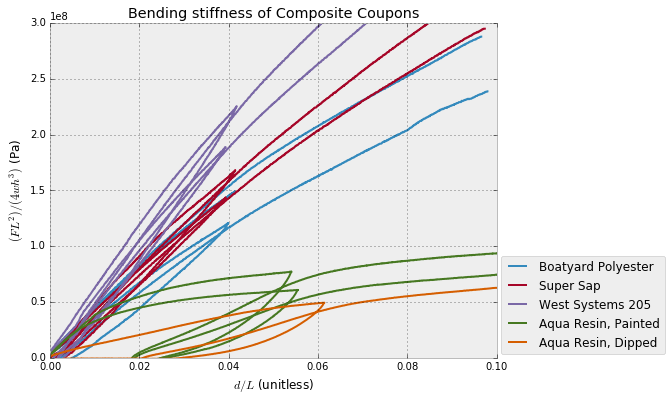### Plotting Specific Bending stiffness¶

In :
figure(figsize=(8,6))
used_labels = []
for record in data:
plot( record['data'][:,1], record['data'][:,2]/record['mass'], c=record['color'], label=record['name'] if record['name'] not in used_labels else '')
used_labels.append(record['name'])
xlim([0,.1])
ylim([0,2.5e7])
#by_label = OrderedDict(zip(gca().get_legend_handles_labels())) #reduce duplicate legend entries
#legend(by_label.values(), by_label.keys())
legend(loc=(1.01,.01))
#legend(loc='lower right')
xlabel('$d/L$ (unitless)')
ylabel('$(FL^2)/(4wh^3)/m$ (Pa/g)')
title('Specific Bending stiffness of Composite Coupons')

Out:
<matplotlib.text.Text at 0x11a1f2490>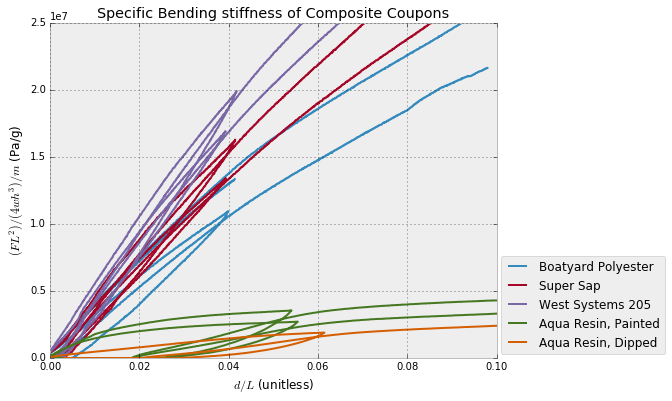## Microscopy for Compaction Method¶

Here we compare a few proposed methods for easy hand layup. First, dry fiber is wet out with Super Sap epoxy. In the first method, coupons are squeegied to remove excess resin and let to cure. In the second, coupons are placed under hydrostatic compaction (a garbage bag). In the third, coupons are placed in a vacuum bag (Space Saver) and put under vacuum. In the fourth, coupons are covered with a perforated bleeder layer, followed by a breather layer (quilting batting), and then placed under vacuum as before. In the final method, coupons are placed between two boards and clamped.

The microscope photographs show approximately 3mm thick samples with back and front illumination. A helpful way to gauge compaction is to look at the distance between fibers running in plane and out of plane.

The resounding message of the microscopy and instron testing is that providing an easy path for resin evacuation is almost more important than compaction force.

### Squeegie¶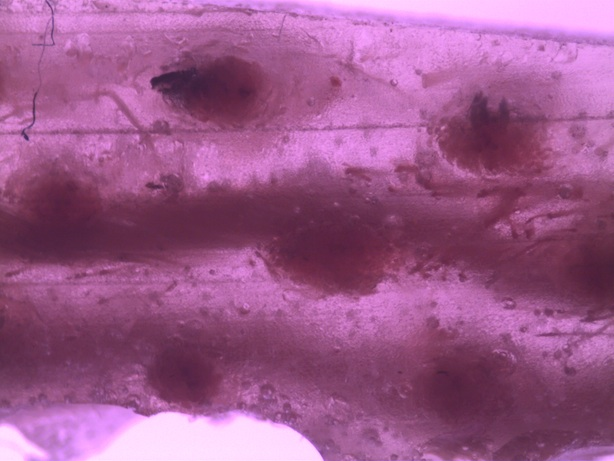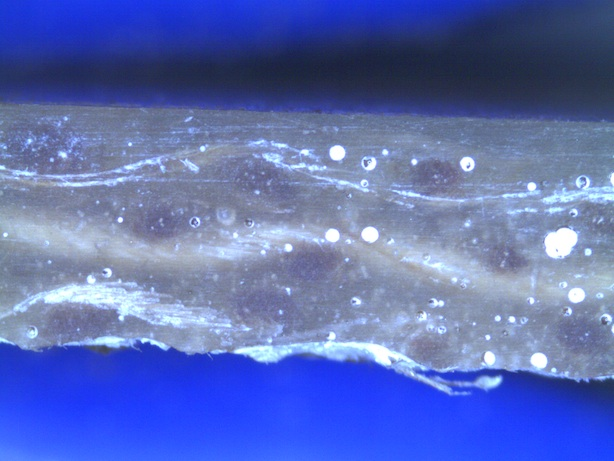### Hydrostatic¶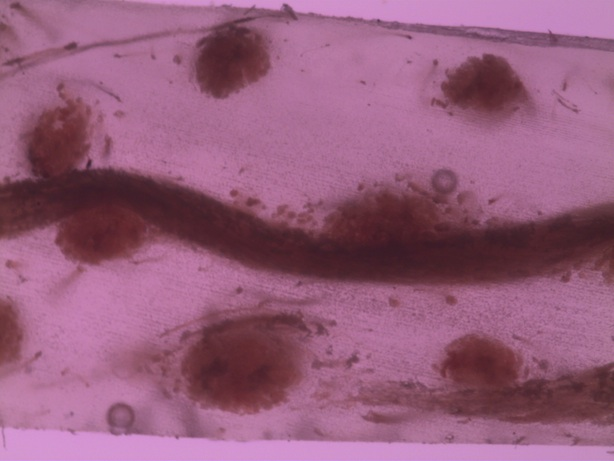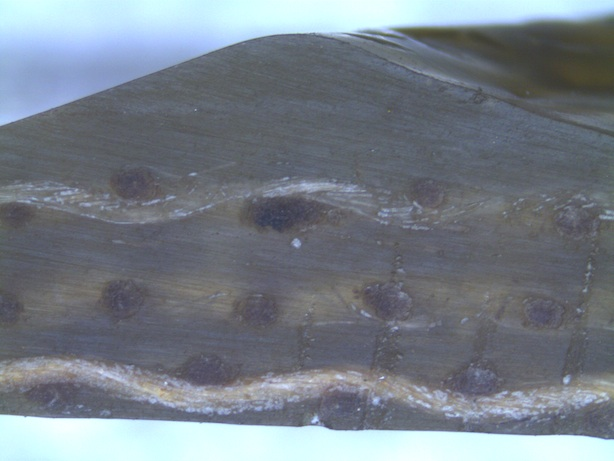### Vacuum without Bleeder/Breather¶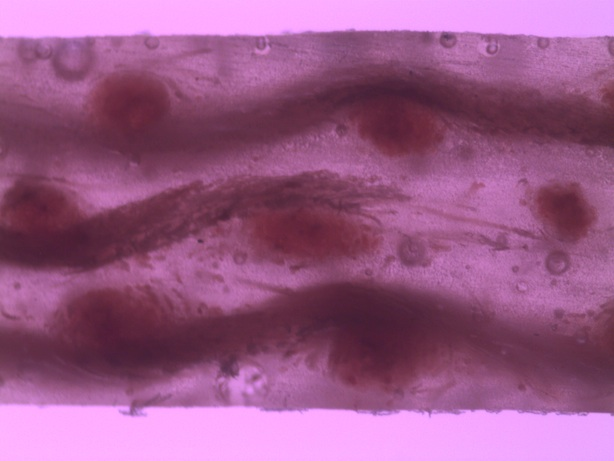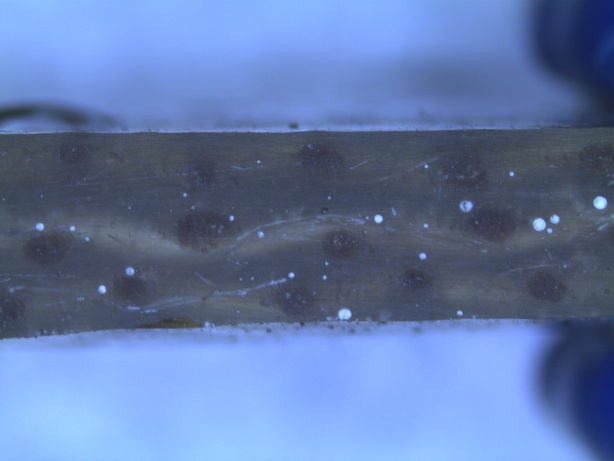### Vacuum with Bleeder/Breather¶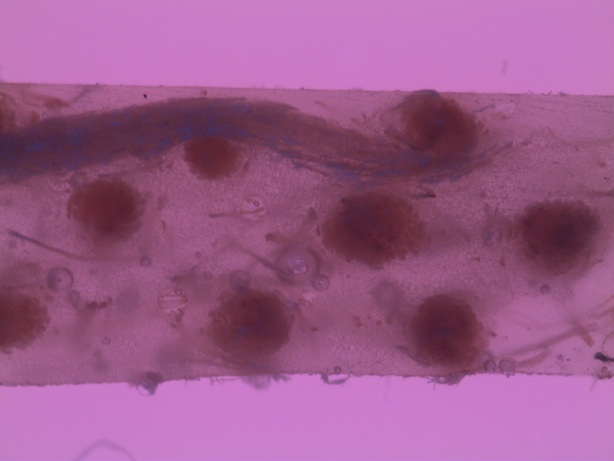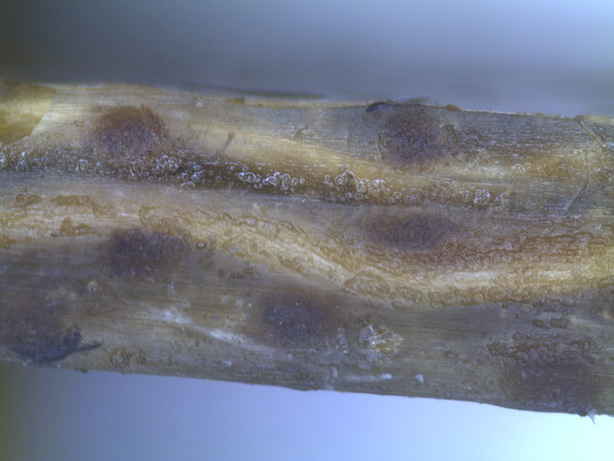### Clamps¶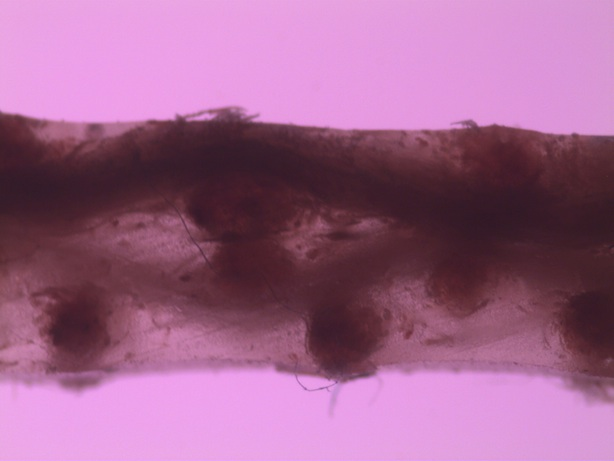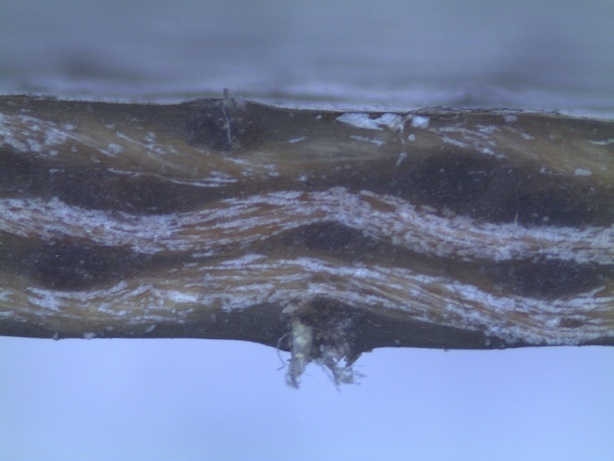### Specific Stiffness vs. Compaction Method¶

In :
labels = [
'Squeegie','Squeegie',
'Hydrostatic','Hydrostatic',
'Vacuum without bleeder','Vacuum without bleeder',
'Vacuum with bleeder','Vacuum with bleeder',
'Clamps','Clamps']
l0 = 75*1e-3 #m, three point bending width
#dimensions to use for density
lengths = 1e-3*asarray([111.38,109.53,110.94,110.37,110.15,112.64,105.83,106.40,111.15,110.24])
widths = 1e-3*asarray([[33.08,33.61],[32.78,33.40],[32.09,32.78],[31.83,32.96],[32.37,32.98],[33.05,33.10],[32.63,34.13],[33.23,33.55],[32.32,33.02],[33.58,32.34]]) #mm
thicknesses = 1e-3*asarray([
[3.76,3.91,4.15],
[3.65,3.95,3.76],
[3.53,4.32,3.71],
[3.98,4.10,4.49],
[3.77,3.94,3.84],
[3.70,4.16,3.93],
[3.29,3.27,3.05],
[2.87,3.03,2.98],
[1.96,2.35,2.42],
[2.23,2.53,2.31],
]) #thickness at end, middle, other end.
masses = [14.96,13.72,16.00,17.30,15.83,16.65,12.67,11.53,8.76,9.61] #grams
#plotting
colors = [0,0,1,1,2,2,3,3,4,4]
fn = lambda i: 'instron-compaction/Specimen_RawData_%d.csv'%(i+1)
data = []
for ind in range(10):
record = {'name': labels[ind],
'mass':masses[ind],
'thickness':sum(thicknesses[ind])/3,
'width':sum(widths[ind])/2,
'length':lengths[ind],
'color':cols[colors[ind]]}
record['volume'] = record['length']*record['width']*record['thickness']
record['density'] = record['mass']/record['volume']
with open(fn(ind), 'r') as f:
spec_data = []
spec_data.append(map(float,l.strip('\n').split(',')))
spec_data = asarray(spec_data)
spec_data[:,1] *= -1 #make positive
spec_data[:,2] *= -1 #make positive
spec_data -= spec_data #subtract initial displacement
spec_data[:,1] *= 1e-3 #scale displacement to meters
spec_data[:,1] *= (1/l0) #scale displacement by l0 to get unitless
spec_data[:,2] *= (l0**2)/(4*record['width']*record['thickness']**3) #scale force to get pressure units
record['data'] = spec_data

data.append(record)

In :
#Bending Stiffness
figure(figsize=(10,8))
used_labels = []
for record in data:
plot( record['data'][:,1], record['data'][:,2], c=record['color'], label=record['name'] if record['name'] not in used_labels else '')
used_labels.append(record['name'])
xlim([0,.11])
ylim([0,4e8])

legend(loc='upper left')
xlabel('$d/L$ (unitless)')
ylabel('Bending Stress $(FL^2)/(4wh^3)$ (Pa)')
title('Bending stiffness of Composite Coupons')

Out:
<matplotlib.text.Text at 0x10d4b2250>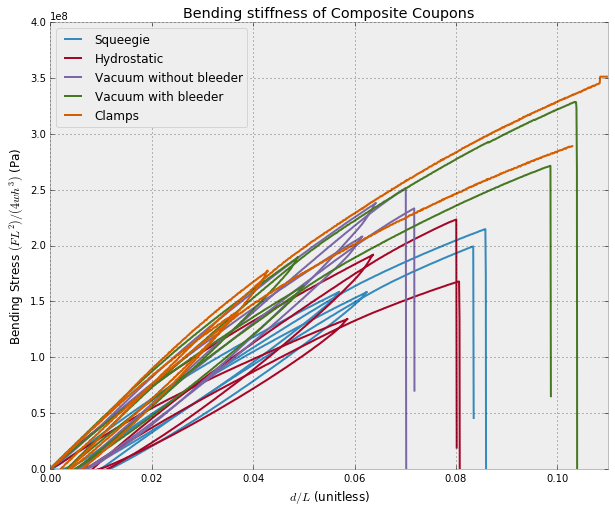In :
#Specific Stiffness
figure(figsize=(10,8))
used_labels = []
for record in data:
plot( record['data'][:,1], record['data'][:,2]/record['density'], c=record['color'], label=record['name'] if record['name'] not in used_labels else '')
used_labels.append(record['name'])
xlim([0,.11])
ylim([0,350])

legend(loc='upper left')
xlabel('$d/L$ (unitless)')
ylabel(r'Specific Bending Stress $(FL^2)/(4wh^3)/\rho$ $(Nm/kg)$')
title('Specific Bending stiffness of Composite Coupons')

Out:
<matplotlib.text.Text at 0x10dc12610>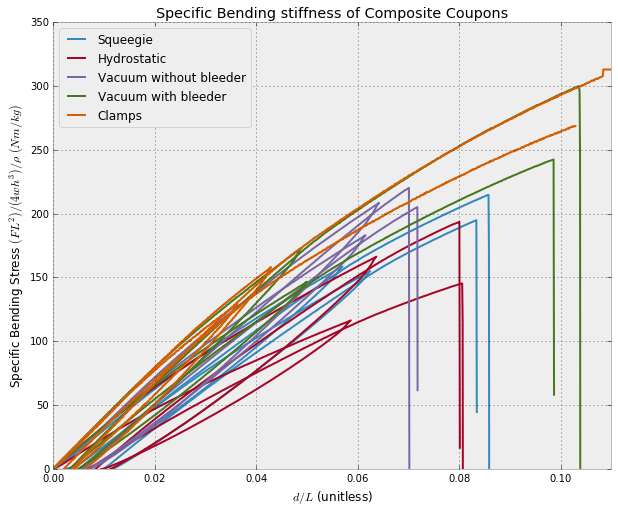## Specific Stiffness vs. Fiber Type¶

We compared carbon fiber cloth with super sap in a vacuum bag, fiberglass cloth with super sap in a vacuum bag, and comingled fiberglass and polypropelyne fibers, thermally consolidated under pressure.

In :
labels = [
'Carbon Super Sap','Carbon Super Sap',
'Fiberglass Super Sap','Fiberglass Super Sap',
'Fiberglass Polypropylene','Fiberglass Polypropylene']
l0 = 75*1e-3 #m, three point bending width
#dimensions to use for density
lengths = asarray([4.58,4.46,4.68,4.53,4.5,4.47])/39.3
widths =  asarray([1.5,1.46,1.51,1.39,1.56,1.57])/39.3
thicknesses = asarray([.066,.061,.113,.123,.040,.044])/39.3 #thickness
masses = [7.3,7.0,14.0,14.3,6.1,6.7] #grams
#plotting
colors = [0,0,1,1,2,2,3,3]
fn = lambda i: 'instron-fiber/Specimen_RawData_%d.csv'%(i+1)
#data = [] #clear data
data = [record for record in data if record['name']=='Vacuum without bleeder'] #keep vacuum from before
for record in data:
record['name'] = 'Burlap Super Sap'
record['color'] = cols
for ind in range(6):
record = {'name': labels[ind],
'mass':masses[ind],
'thickness':thicknesses[ind],
'width':widths[ind],
'length':lengths[ind],
'color':cols[colors[ind]]}
record['volume'] = record['length']*record['width']*record['thickness']
record['density'] = record['mass']/record['volume']
with open(fn(ind), 'r') as f:
spec_data = []
spec_data.append(map(float,l.strip('\n').split(',')))
spec_data = asarray(spec_data)
spec_data[:,1] *= -1 #make positive
spec_data[:,2] *= -1 #make positive
spec_data -= spec_data #subtract initial displacement
spec_data[:,1] *= 1e-3 #scale displacement to meters
spec_data[:,1] *= (1/l0) #scale displacement by l0 to get unitless
spec_data[:,2] *= (l0**2)/(4*record['width']*record['thickness']**3) #scale force to get pressure units
record['data'] = spec_data

data.append(record)
def smooth(y, box_pts):
box = np.ones(box_pts)/box_pts
y_smooth = np.convolve(y, box, mode='same')
return y_smooth

In [ ]:
#Bending Stiffness
figure(figsize=(10,8))
used_labels = []
for record in data:
plot( record['data'][:,1], smooth(record['data'][:,2],5), c=record['color'], label=record['name'] if record['name'] not in used_labels else '')
used_labels.append(record['name'])
#xlim([0,.11])
ylim([0,4e8])

legend(loc='upper left')
xlabel('$d/L$ (unitless)')
ylabel('Bending Stress $(FL^2)/(4wh^3)$ (Pa)')
title('Bending stiffness of Composite Coupons')

In :
#Specific Stiffness
figure(figsize=(10,8))
used_labels = []
for record in data:
plot( record['data'][:-7,1], smooth(record['data'][:,2],7)[:-7]/record['density'], c=record['color'], label=record['name'] if record['name'] not in used_labels else '')
used_labels.append(record['name'])
xlim([0,.11])
ylim([0,600])

legend(loc='upper left')
xlabel('$d/L$ (unitless)')
ylabel(r'Specific Bending Stress $(FL^2)/(4wh^3)/\rho$ $(Nm/kg)$')
title('Specific Bending stiffness of Composite Coupons')

Out:
<matplotlib.text.Text at 0x10e98a090>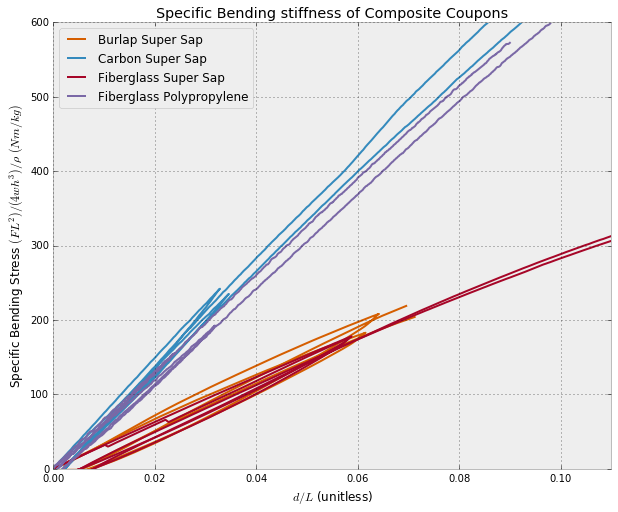In [ ]: